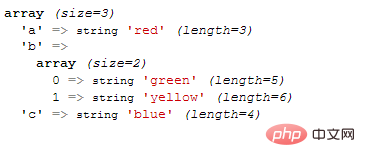# php数组合并怎么不去重复array_merge_recursive() 函数把一个或多个数组合并为一个数组。

`array_merge_recursive(array1,array2,array3...)`

```<?php
\$a1=array("a"=>"red","b"=>"green");
\$a2=array("c"=>"blue","b"=>"yellow");
\$arr=array_merge_recursive(\$a1,\$a2);
var_dump(\$arr);
?>```1/1